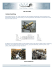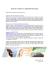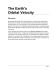# HW9

## Transcription

HW9
```Mech 301 Fluid Mechanics
HW9
1. (M-P6.93) An incompressible, Newtonian fluid flows
steadily between two infinitely long, concentric cylinders as
shown in the sketch. The outer cylinder is fixed, but the
inner cylinder moves with a longitudinal velocity V0 as
shown. For what value of V0 will the drag on the inner
cylinder be zero? Assume that the flow is laminar, axisymmetric
and fully developed.
2. (P4.92 in the book) Modify the analysis of Fig. 4.13 to find the
velocity vθ when the inner cylinder is fixed and the outer cylinder
rotates at angular velocity Ω0. May this solution be added to Eq.
(4.140) to represent the flow caused when both inner and outer
3. (P4.97 in the book) SAE 10 oil at 20°C flows between
parallel plates 8 mm apart, as in Fig. 4.97. A mercury
manometer, with wall pressure taps 1 m apart, registers a
6-cm height, as shown. Estimate the flow rate of oil for this
condition.
4. (Problem P5.72 in the Book) The power P generated by a certain windmill design depends upon its
diameter D, the air density , the wind velocity V, the rotation rate , and the number of blades n.
(a) Write this relationship in dimensionless form. A model windmill, of diameter 50 cm, develops
2.7 kW at sea level when V  40 m/s and when rotating at 4800 rev/min. (b) What power will be
developed by a geometrically and dynamically similar prototype, of diameter 5 m, in winds of 12
m/s at 2000 m standard altitude? (c) What is the appropriate rotation rate of the prototype?
5. (M2-Q3-2004) Consider an air bubble with radius R, density  a , and
viscosity  a rising in water of density  w , and viscosity  w solely
due to buoyancy as sketched. Assuming that the velocity of the
bubble is given by
U  f ( R,  ,  a ,  w , g ,  )
where    w   a , g is the gravitational acceleration, and  is the
surface tension.
a) Using the Buckingham Pi-theorem, write this equation in
dimensionless form.
b) It is determined experimentally that the velocity of an air bubble of radius R = 0.1 cm
is 3.27 m/s. Is it possible to predict the velocity of a same-size air bubble rising in
glycerin?
(  w  10 3 kg / m 3 ,  w  10 3 kg / m  s,  a  1.26kg / m 3 ,  a  1.8 x10 5 kg / m  s,
 glycerin  1260kg / m 3 ,  glycerin  1.49kg / m  s,  air water  0.073N / m,  air glycerin  0.0633N / m)
```

### MAE 2600 (FS2014) Homework #18 Name: H18-1.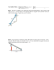### Throw a ball up and it will fall down 1) The force of gravity pulls the### Solving Literal Equations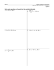### Science 9 chapter 8 intext page 102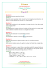### Speed, Velocity, and Acceleration Worksheet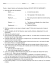### Assignment 3 cover sheet Student name: Student number: Tutor name: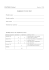### Bubble Trouble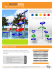### Chemistry – Unit 1.2 Worksheet 3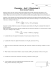### Chapter 5 Review Physics 11 1.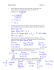### CCS Cylincer Reclothing - Card Clothing & Services